# Texas Go Math Grade 5 Lesson 12.4 Answer Key Apply Volume Formulas

Refer to our Texas Go Math Grade 5 Answer Key Pdf to score good marks in the exams. Test yourself by practicing the problems from Texas Go Math Grade 5 Lesson 12.4 Answer Key Apply Volume Formulas.

## Texas Go Math Grade 5 Lesson 12.4 Answer Key Apply Volume Formulas

Unlock the problem

Mike is making a box to hold his favorite DVDs. The length of the box is 7 inches, the width is 5 inches and the height is 3 inches. What is the volume of the box Mike is making?

One Way Use length, width, and height.

You can use a formula to find the volume of a rectangular prism.
Volume = length × width × height
V = l × w × h
Step 1
Identify the length, width, and height of the rectangular prism.Step 2
Multiply the length by the width.
7 × 5 = 35
Step 3
Multiply the product of the length and width by the height.
35 × 3 = 105
So,
The volume of Mike’s DVD box is 105 cubic inches.

You have learned one formula for finding the volume of a rectangular prism.
You can also use another formula.
Volume = Base area × height
V = B × h
B = area of the base shape,
h = height of the solid figure.

Another Way Use the area of the base shape and height.
Emilio’s family has a sandcastle kit. The kit includes molds for several solid figures that can be used to make sandcastles. One of the molds is a rectangular prism-like the one shown at the right. How much sand will it take to fill the mold?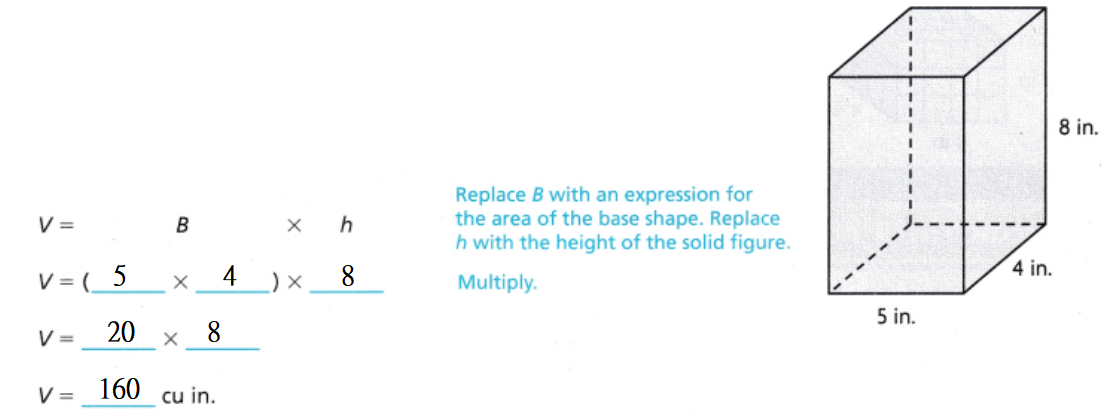So,
It will take 160 cubic inches of sand to fill the rectangular prism mold.

Share and Show

Find the volume.

Question 1.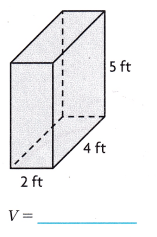The given figure is: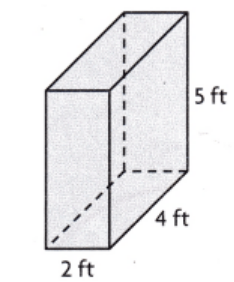Now,
From the given figure,
We can observe that
The volume of the given figure = Length × Width × Height
= 2 × 4 × 5
= 40 ft³
Hence, from the above,
We can conclude that
The volume of the given figure is: 40 ft³The given figure is: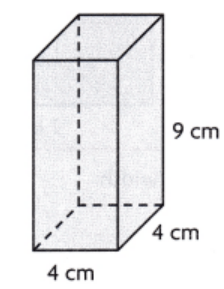Now,
From the given figure,
We can observe that
The volume of the given figure = Length × Width × Height
= 4 × 4 × 9
= 144 cm³
Hence, from the above,
We can conclude that
The volume of the given figure is: 144 cm³

Problem Solving

H.O.T. Algebra Find the unknown measurements.

Question 3.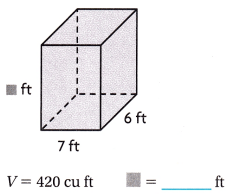The given figure is:Now,
From the given figure,
We can observe that
The volume of the given figure = Length × Width × Height
Now,
Let the unknown measurement be x
So,
420 = 7 × 6 × x
x = $$\frac{420}{42}$$
x = 10 ft
Hence, from the above,
We can conclude that
The value of the unknown measurement is: 10 ft

Question 4.The given figure is: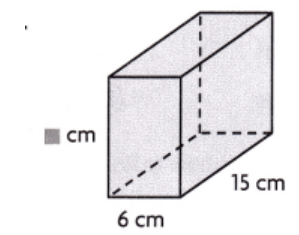Now,
From the given figure,
We can observe that
The volume of the given figure = Length × Width × Height
Now,
Let the unknown measurement be x
So,
900 = 15 × 6 × x
x = $$\frac{900}{90}$$
x = 10 cm
Hence, from the above,
We can conclude that
The value of the unknown measurement is: 10 cm

Write Math Describe the difference between area and volume.
The area is the measurement of the region covered by any two-dimensional geometric shapes. The volume is the space occupied by the three-dimensional object. Volume is measured for 3D(solid) figures. The area is measured in two dimensions i.e length and breadth

Problem Solving

Question 6.
Apply The Jade Restaurant has a large aquarium on display in its lobby. The base of the aquarium is 5 feet by 2 feet. The height of the aquarium is 4 feet. How many cubic feet of water is needed to completely fill the aquarium?
It is given that
The Jade Restaurant has a large aquarium on display in its lobby. The base of the aquarium is 5 feet by 2 feet. The height of the aquarium is 4 feet
So,
The number of cubic feet of water needed to completely fill the aquarium = (The Base of the aquarium) × (The height of the aquarium)
= (5 × 2) × 4
= 40 ft³
Hence, from the above,
We can conclude that
The number of cubic feet of water needed to completely fill the aquarium is: 40 ft³

Question 7.
H.O.T. Multi-Step The Pearl Restaurant put a larger aquarium in its lobby. The base of their aquarium is 6 feet by 3 feet, and the height is 4 feet. How many more cubic feet of water does the Pearl Restaurant’s aquarium hold than the Jade Restaurant’s aquarium?
From Exercise 6,
We know that,
The number of cubic feet of water needed to completely fill the aquarium is: 40 ft³
Now,
It is given that
The Pearl Restaurant put a larger aquarium in its lobby. The base of their aquarium is 6 feet by 3 feet, and the height is 4 feet.
So,
The number of cubic feet of water the Pearl restaurant’s aquarium hold than the Jade Restaurant’s aquarium = (6 × 3) × 4 – 40
= (18 × 4) – 40
= 72 – 40
= 32 ft³
Hence, from the above,
We can conclude that
The number of cubic feet of water the Pearl restaurant’s aquarium hold than the Jade Restaurant’s aquarium is: 32 ft³

Question 8.
H.O.T. Eddie measured his aquarium using a small fish food box. The box has a base area of 6 inches and a height of 4 inches. Eddie found that the volume of his aquarium is 3,456 cubic inches. How many boxes of fish food could fit in the aquarium? Explain your answer.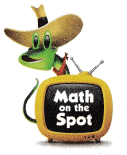It is given that
Eddie measured his aquarium using a small fish food box. The box has a base area of 6 inches and a height of 4 inches. Eddie found that the volume of his aquarium is 3,456 cubic inches
So,
The volume of the aquarium = (The Base area of the aquarium) × (The number of boxes of fish food could fit in the aquarium)
So,
3,456 = (6 × 4) × (The number of boxes of fish food could fit in the aquarium)
The number of boxes of fish food could fit in the aquarium = $$\frac{3,456}{24}$$
The number of boxes of fish food could fit in the aquarium = 12
Hence, from the above,
We can conclude that
The number of boxes of fish food could fit in the aquarium is: 12 boxes

Question 9.
A cardboard box has a length of 12 inches, a width of 7 inches, and a height of 4 inches. What is the volume of the box?
(A) 23 cubic inches
(B) 84 cubic inches
(C) 46 cubic inches
(D) 336 cubic inches
It is given that
A cardboard box has a length of 12 inches, a width of 7 inches, and a height of 4 inches
So,
The volume of the box = Length × Width × Height
= 12 × 7 × 4
= 336 inches³
Hence, from the above,
We can conclude that
The volume of the box is:Texas Go Math Grade 5 Volume Pdf Volume Formula Question 10.
Which expression shows how to find the volume of the following prism?(A) 30 × 3
(B) 6 + 6 + 5 + 5 + 3 + 3
(C) 6 × 5 × 3 × 6 × 5 × 3
(D) 6 × 5
The given figure is:Now,
From the given figure,
We can observe that
The length of the given figure is: 6 units
The width of the given figure is: 5 units
The height of the given figure is: 3 units
So,
The volume of the given figure = Length × Width × Height
= (6 × 5) × 3
= 30 × 3
= 90 sq. units
Hence, from the above,
We can conclude that
The volume of the given figure is: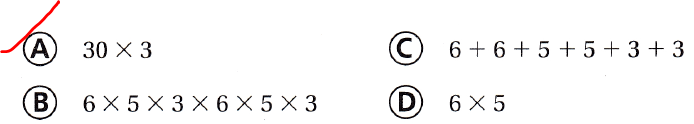Question 11.
Multi-Step Jumana built these prisms out of cube-shaped blocks. How many blocks did she use?
(A) 120 blocks
(B) 84 blocks
(C) 21 blocks
(D) 1,440 blocks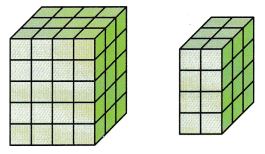It is given that
Jumana built these prisms out of cube-shaped blocks
Now,
The given figures are:Now,
From the given figures,
We can observe that
The total number of blocks did Jumana used = (4 × 3 × 5) + (2 × 3 × 4)
= 60 + 24
= 84 blocks
Hence, from the above,
We can conclude that
The total number of blocks did Jumana used is: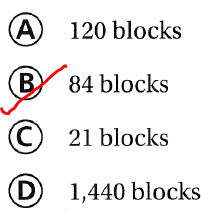Texas Test Prep

Grade 5 Lesson 12.4 Find Volume Using Formulas Answer Key Question 12.
Adam stores his favorite CDs in a box like the one shown below. What is the volume of the box?
(A) 750 cubic centimeters
(B) 150 cubic centimeters
(C) 1,050 cubic centimeters
(D) 1,150 cubic centimetersIt is given that
Adam stores his favorite CDs in a box like the one shown below
Now,
The given figure is:Now,
According to the given information,
The volume of the box = 15 × 10 × 7
= 1,050 cm³
Hence, from the above,
We can conclude that
The volume of the box is:### Texas Go Math Grade 5 Lesson 12.4 Homework and Practice Answer Key

Find the unknown measurement.

Question 1.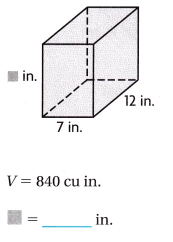The given figure is: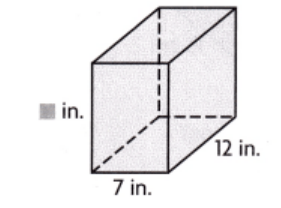Now,
From the given figure,
We can observe that
The volume of the given figure = Length × Width × Height
Now,
Let the unknown measurement be x
So,
840 = 12 × 7 × x
x = $$\frac{840}{84}$$
x = 10 in.
Hence, from the above,
We can conclude that
The value of the unknown measurement is: 10 in.

Question 2.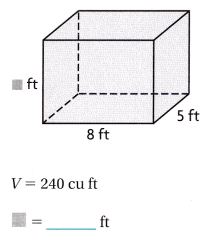The given figure is:Now,
From the given figure,
We can observe that
The volume of the given figure = Length × Width × Height
Now,
Let the unknown measurement be x
So,
240 = 8 × 5 × x
x = $$\frac{240}{40}$$
x = 6 ft
Hence, from the above,
We can conclude that
The value of the unknown measurement is: 6 ft

Grade 5 Lesson 12.4 Find Volume Using Formulas Question 3.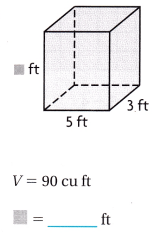The given figure is: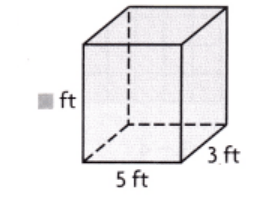Now,
From the given figure,
We can observe that
The volume of the given figure = Length × Width × Height
Now,
Let the unknown measurement be x
So,
90 = 3 × 5 × x
x = $$\frac{90}{15}$$
x = 6 ft
Hence, from the above,
We can conclude that
The value of the unknown measurement is: 6 ft

Question 4.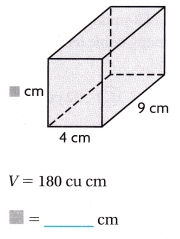The given figure is: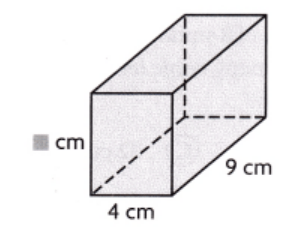Now,
From the given figure,
We can observe that
The volume of the given figure = Length × Width × Height
Now,
Let the unknown measurement be x
So,
180 = 4 × 9 × x
x = $$\frac{180}{36}$$
x = 5 cm
Hence, from the above,
We can conclude that
The value of the unknown measurement is: 5 cm

Question 5.The given figure is:Now,
From the given figure,
We can observe that
The volume of the given figure = Length × Width × Height
Now,
Let the unknown measurement be x
So,
500 = 10 × 5 × x
x = $$\frac{500}{50}$$
x = 10 cm
Hence, from the above,
We can conclude that
The value of the unknown measurement is: 10 cm

Question 6.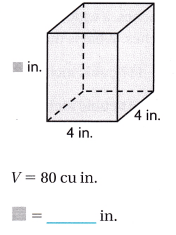The given figure is: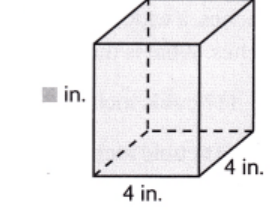Now,
From the given figure,
We can observe that
The volume of the given figure = Length × Width × Height
Now,
Let the unknown measurement be x
So,
80 = 4 × 4 × x
x = $$\frac{80}{16}$$
x = 5 in.
Hence, from the above,
We can conclude that
The value of the unknown measurement is: 5 in.

Problem Solving

Question 7.
At the community center, athletic gear is stored in a large locker. The locker floor is 10 feet by 9 feet. The height of the locker is 8 feet. How much space is in the locker?
It is given that
At the community center, athletic gear is stored in a large locker. The locker floor is 10 feet by 9 feet. The height of the locker is 8 feet
So,
According to the given information,
The amount of space that is present in the locker = (Base Area) × (The height of the locker)
= 10 × 9 × 8
= 720 ft²
Hence, from the above,
We can conclude that
The amount of space that is present in the locker is: 720 ft²

Question 8.
A storage locker is 15 ft long, 2 ft wide, and 4 ft high. How many boxes that are 3 ft long, 2 ft wide, and 1 ft high can the locker lit?
It is given that
A storage locker is 15 ft long, 2 ft wide, and 4 ft high
Now,
According to the given information,
The volume of the storage locker = 15 × 2 × 4
= 120 ft²
Now,
The volume of the box = 3 × 2 × 1
= 6 ft²
So,
The number of boxes can the locker fit = $$\frac{120}{6}$$
= 20 boxes
Hence, from the above,
We can conclude that
The number of boxes can the locker fit is: 20 boxes

Lesson Check

Texas Test Prep

Question 9.
Which expression shows how to find the volume of the prism shown below?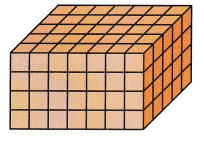(A) 7 × 5 + 4
(B) 35 × 4
(C) 7 + 7 + 7 + 7 × 5
(D) 7 + 5 × 4
The given figure is:Now,
From the given figure,
We can observe that
The length of the given figure is: 7 units
The width of the given figure is: 5 units
The height of the given figure is: 4 units
So,
The volume of the given figure = Length × Width × Height
= 7 × 5 × 4
= 35 × 4
= 140 sq. units
Hence, from the above,
We can conclude that
The volume of the given figure is: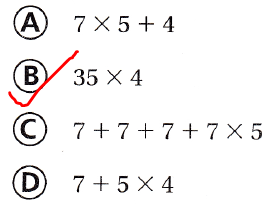Jack builds a box for a birdhouse like the one shown below.What is the volume of the box?
(A) 1,426 cubic centimeters
(B) 288 cubic centimeters
(C) 1,536 cubic centimeters
(D) 140 cubic centimeters
It is given that
Jack builds a box for a birdhouse like the one shown below.
Now,
The given figure is:So,
The volume of the box = Length × Width × Height
= 12 × 8 × 16
= 192 × 8
= 1,536 cm²
Hence, from the above,
We can conclude that
The volume of the box is: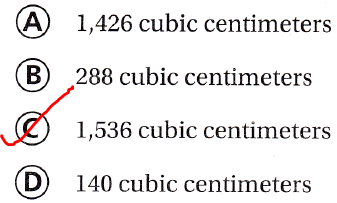Question 11.
A rectangular cake pan has a length of 13 inches, a width of 9 inches, and a height of 3 inches. What is the volume of the cake pan?
(A) 117 cubic inches
(B) 351 cubic inches
(C) 120 cubic inches
(D) 66 cubic inches
It is given that
A rectangular cake pan has a length of 13 inches, a width of 9 inches, and a height of 3 inches
So,
According to the given information,
The volume of the cake pan = Length × Width × Height
= 13 × 9 × 3
= 13 × 27
= 351 in.³
Hence, from the above,
We can conclude that
The volume of the cake pan is:Question 12.
Jada stores her winter clothes in a wooden chest with a base area of 8 square feet and a height of 2 feet. How many cubic feet of space does the chest contain?
(A) 128 cubic feet
(B) 10 cubic feet
(C) 32 cubic feet
(D) 16 cubic feet
It is given that
Jada stores her winter clothes in a wooden chest with a base area of 8 square feet and a height of 2 feet
Now,
According to the given information,
The number of cubic feet of space the chest contained = (The Base area) × Height
= 8 × 2
= 16 ft³
Hence, from the above,
We can conclude that
The number of cubic feet of space the chest contained is:Question 13.
Multi-Step Malaya packs two small suitcases each with a length of 2 feet, a width of 2 feet, and a height of 1 foot. What is the difference between their combined volume and the volume of one large suitcase with a length of 4 feet, a width of 3 feet, and a height of 2 feet?
(A) 20 cubic feet
(B) 16 cubic feet
(C) 19 cubic feet
(D) 14 cubic feet
It is given that
Malaya packs two small suitcases each with a length of 2 feet, a width of 2 feet, and a height of 1 foot
Now,
According to the given information,
The volume of the two small suitcases = 2 × 2 × 2 × 1
= 8 ft³
Now,
The volume of tone large suitcase = 4 × 3 × 2
= 24 ft³
So,
The difference between the combined volume of two small suitcases and the volume of one large suitcase = 24 – 8
= 16 ft³
Hence, from the above,
We can conclude that
The difference between the combined volume of two small suitcases and the volume of one large suitcase is: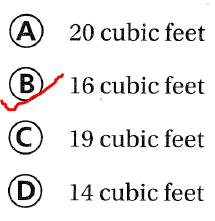Question 14.
Multi-Step Candace and Trevor built the prisms shown below out of cube-shaped blocks.How many more blocks were used in the prism with the greater volume?
(A) 24 blocks
(B) 48 blocks
(C) 12 blocks
(D) 36 blocks
It is given that
Candace and Trevor built the prisms shown below out of cube-shaped blocks.
Now,
The given figures are:Now,
From the above figures,
We can observ ethat
The number of more blocks used in the greater prism = (4 × 3 × 4) – (4 × 2 × 3)
= 48 – 24
= 24 blocks
Hence, from the above,
We can conclude that
The number of more blocks used in the greater prism is:Scroll to Top